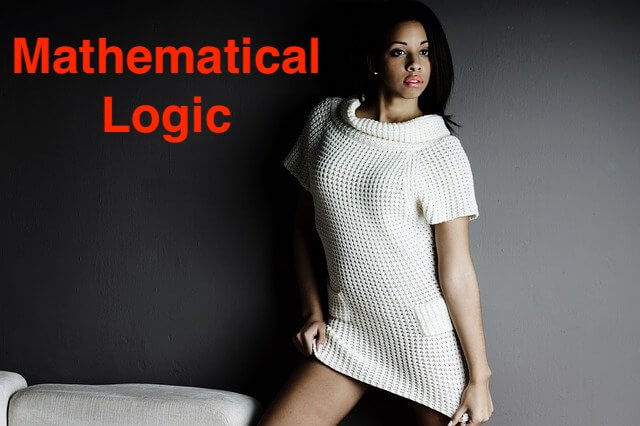### Determine the truth value of the following statement. If 9 > 1 then x2 − 2x + 1 = 0 for x = 1

#### QUESTION

Miscellaneous Exercise 1 | Q 4.06 | Page 32

Determine the truth value of the following statement.

If 9 > 1 then x2 − 2x + 1 = 0 for x = 1

#### SOLUTION

Let p : 9 > 1
q : x2 – 2x + 1 = 0 for x = 1

The truth values of p and q are T and T respectively. The given statement in symbolic form is p → q.

∴ p → q ≡ T → T ≡ T

∴ Truth value of the given statement is T.